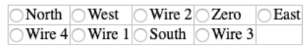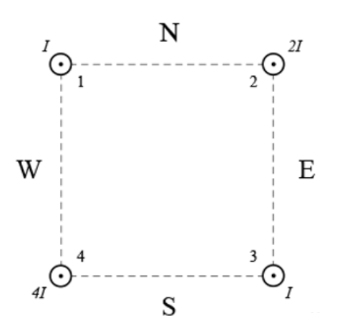# Problem: Four current carrying wires are arranged in the corners of a square as shown in the picture.Magnetic field in the center of the square is directed towards:

###### FREE Expert Solution

Magnetic field around a wire carrying current:

$\overline{){\mathbf{B}}{\mathbf{=}}\frac{{\mathbf{\mu }}_{\mathbf{0}}\mathbf{i}}{\mathbf{2}\mathbf{\pi }\mathbf{r}}}$

Right-hand rule: Thumb points in the direct current, fingers curl in the direction of the magnetic field.

At the center of the square, r from each wire is equal. However, the current in each wire is different.

95% (473 ratings)###### Problem Details

Four current carrying wires are arranged in the corners of a square as shown in the picture.

Magnetic field in the center of the square is directed towards: